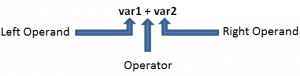Note: I do focus on C++ in this text.

Operator overload is a very important and useful feature within C++. Lets say you have developed a new class which shall be used in a larger system. Your class has methods for printing its content to the console, to increment its inner value etc. The problem with that is that the usage is not intuitive.

```myClass var1=4;
myClass var2=5;

myClass result=0;

result.print();```

Its way more comfortable if one can use the class with operators.

```myClass var1=4;
myClass var2=5;

myClass result=0;

result=var1+var2;
cout<<result;```
##### Basic Structure

First of all, a operator overload is just a link to predefined method. So basically you define which method to be invoked if your class is called with an operator.

The structure of a call with operator is the following:Also we do have a return value for the operation which can be used to fetch the new value.

We can see that the content of the operands is not changed (so we should make them const) but we give back a instance with the new value which then might be used by another method:

```myClass result2;

result2.set(var1+var2);```
##### Member/non-member implementation

We have to decide whether we want to implement the operator overload as member or non-member overload.

Member

If we implement the overload as a member than we do it like we would implement a method, we simply extend our class. Also we do not have a left operand anymore as it is known already, it is the class itself.

Lets assume our Class has a member "m_value" which we want to add.

`myClass operator+(myClass & right);`

Implementation:

```myClass operator+(myClass & right) {
return myClass (m_value+right.m_value);
}```

Non-Member

However, sometimes it is not possible to extend a class as we are not able to do so (extending cout etc). Instead of extending the left operand class we also can implement non-member operand overloads. Basically the idea is that we tell the compiler that whenever a condition of certain classes with a certain operator is found to invoke a defined method.

```myClass operator+(myClass & left,myClass & right) {
return myClass (left.m_value+right.m_value);
}```

As you can see we now have both operands as parameters. The problem with non-member operator overload is that it might want to access private attributes. Of course it cant because its just a function which is called outside the class. To make the private attributes accessible we need to declare the function as a "friend".

`friend myClass operator+(myClass & left,myClass & right);`

##### Operators

Need to be member methods (by definition):
= (assignment)
[] (array subscription)
-> (member access)
() (function call)

Need to be non member functions if used with cout cerr etc.  (wherever the left operand can not be modified) :
<<
>>

All others can be either member or non-member methods (+, -, *, /, %  +=, -=, *=, /=, %=, &=, |=, ^= etc)